# Cassini(an) oval

## quartic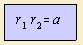Given two foci F1(-1,0) and F2(1,0), you can distinguish two polar coordinates, with respect to each of the foci. The curve for which the product of this two polar radii is a constant, is the Cassinian oval 1).
The curve can be generalized to the Cassinian curve.

The French astronomer Giovanni Dominico Cassini (1625-1712) found the curves in 1680, while attempting to describe the movement of the earth relative to the sun. He believed the orbit of the earth was a cassinoid, with the sun in one focus.
Malfatti studied the curve in 1781.

For the curve the product of distances to the two focal points is a constant. This definition resembles the definition of the ellipse, with a product instead of an addition. That's why the curve has also been given the name of the Cassini(an) ellipse. The curve is also named a cassinoid.

The name Cassini has been given to the pilotless spaceship that is right now on his way to the planet Saturn.

The curve is a bicircular quartic 2), and an anallagmatic curve
In polar coordinates the curve is written as: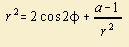The value of the variable named a determines the form of the oval: for a > 1, we see one curve, for a < 1 two egg-shaped forms.
For a  < 2, the oval is squeezed in the middle, for a > 2, the curve goes towards a circle.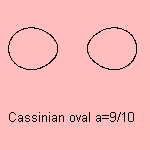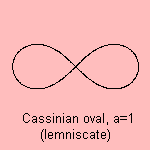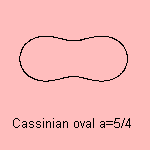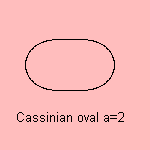The curve is a spiric section, for which the distance from the plane that forms the curve to the axis of the torus is equal to its inner radius.
For a=4 the curve is a Mandelbrot lemsniscate (n=2).

When the variable a is equal to one, the last term in the polar formula vanishes: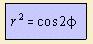with (0, ± 1/√2) as foci.

The resulting curve is the lemniscate or lemniscate of Bernoulli. In 1694 Jacob Bernoulli (1654-1705) wrote an article in Acta Eruditorum about the curve, and he gave it the name of 'lemniscus' 3). He didn't know that 14 years earlier Cassini had already described the more general case of the Cassini ovals.
More properties of the lemniscate were found by Fagnano in 1750.

Research of Gauss and Euler on the length of the arc of the curve led to the elliptic functions.
In 1797 Gauss wrote in his diary: "lemniscata geometrice in quinque partes dividitur", describing his discovery how do divide the lemniscate in five equal parts by ruler and compass.
Knowing nothing about this diary, Abel found this himself, from the remark of Gauss in his Disquisitiones Arithmeticae about the broadening of the principles of the polygon-construction laws to transcendental functions.

Abel formulated his findings more general as the following theorem:
The lemniscate can be divided into n equal parts with ruler and compass if n = 2a p1 p2 .. pi where the pi are distinct Fermat primes 4).

A Lemniscate constant L appears, which has the same meaning as the p for the circle: 2 L is the length of the lemniscate's arc. L is about 2.6221 and can also be written as ˝Γ2(Ľ)/√2π, using the gamma function.
For this calculation often the following formula for the lemniscate is used: ds = dr / √(1 - r4).

Because the curve is the inverse of an equilateral hyperbola, it is also called the hyperbolic lemniscate. In fact, the curve is also the pedal of this hyperbola variant.

The lemniscate can be seen as a special case of the:

• hippopede
• sinusoidal spiral
• generalized cissoid
The lemniscate is the cissoid of two equal circles, where the distance from the center to the center of the circle is √2 times the circle's radius.
• Watt's curve: the length of the rod and the distance between the circle's centers are equal, and the length of the rod is √2 times the radius of the circle)

Besides:

• the curve is the inverse of the rectangular hyperbola
• the curve is the envelope of circles with their centers on a rectangular hyperbola where each circle has a point on the hyperbola's center

Maybe the infinity sign ∞ has been derived from the lemniscate, as this curve is also going round infinitely. Lemniscate is also the name of the longest composition of Western music, made by Simeon ten Holt (30 hours).
A lemniscate is a nice logo, e.g. used by the Dutch publisher 'Lemniscaat' (well-known by his child books).

A three-dimensional variation on the lemniscate is the Möbius strip.

notes

1) In Italian: ovali di Cassini, or ovali cassiniane

2) with Cartesian equation: ((x+1)2+y2)((x-1)2+y2)=a2 which leads to:

(x2+y2)2 + 2x2 - 2y2 = b, with b=a2 -1

3) Lemniskos (Gr.) = ribbon

4) See Rosen 1981 p. 387-395.